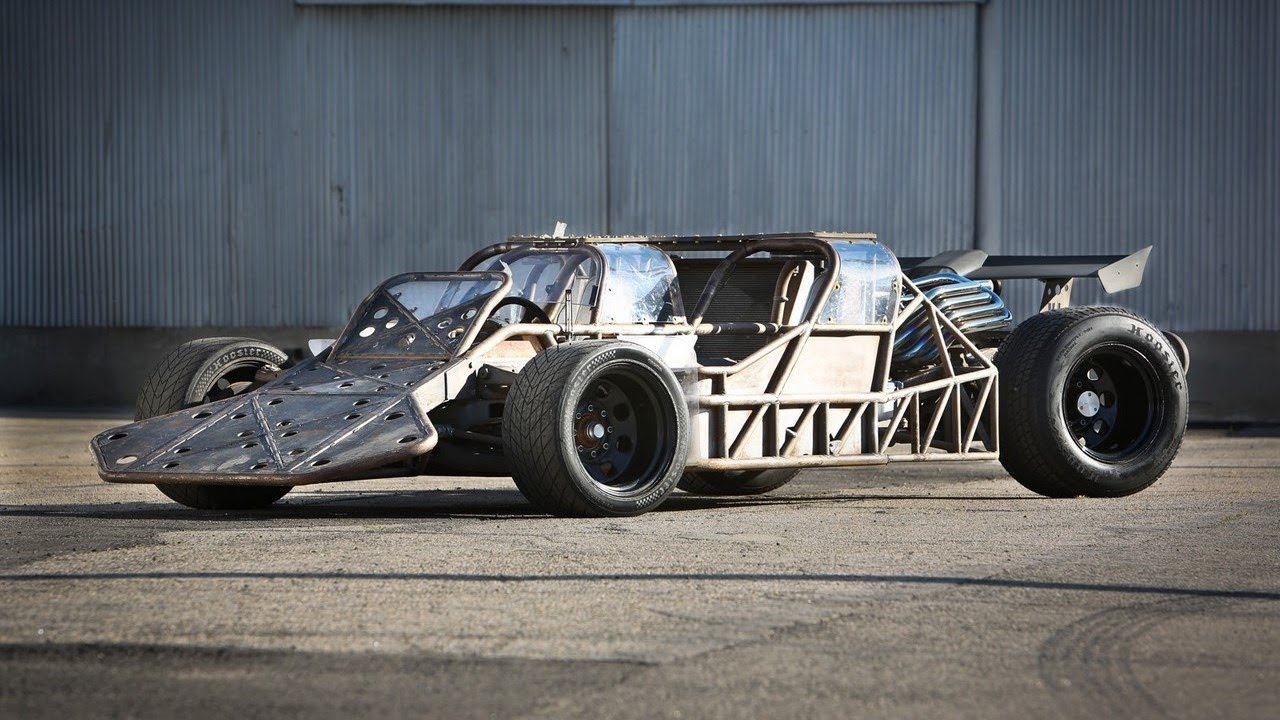Classical Mechanics

# Intensive and extensive propertiesA $$4$$-wheel car stands still with the static force of friction $$f_s.$$ If we attach $$2$$ more wheels on the car, what is the new force of friction? Assume that all the tires have the same coefficient of friction with the ground, and neglect the weights of the wheels.

A $$7.0 \text{ kg}$$ sample of metal with a specific heat of $$0.2 \text{ kJ/kg}^\circ\text{C}$$ is supplied with $$42.0 \text{ kJ}$$ of heat and has its temperature raised to $$48.0 ^\circ \text{C} .$$ Find the initial temperature of the metal.

If helium gas fills a spherical balloon of radius $$16.0 \text{ cm}$$ at $$25.0 ^\circ\text{C}$$ and $$1.013 \times 10^5 \text{ N/m}^2,$$ what is the approximate total kinetic energy of the helium atoms in the balloon?

The value of the Boltzmann constant $$k_B$$ is $$1.38 \times 10^{-23} \text{ J/K}$$ and the value of the universal gas constant $$R$$ is $$8.31 \text{ J/mol}\cdot\text{K}.$$

If the temperature of a liquid is increased by $$10 ^\circ \text{C},$$ its density decreases by $$2 \%.$$ Find the coefficient of volume expansion of the liquid.

Assume that we have found a new multicellular species. During the research of that species, it is found that one cell has $$300,000$$ base pairs of DNA. If a single individual of the new species has about $$25,000$$ cells, what is the total number of base pairs of DNA in its whole body?

×

Problem Loading...

Note Loading...

Set Loading...